# H2CO lewis structure, molecular geometry, polarity, bond angle, hybridization

Home  > Chemistry Article > H2CO lewis structure and its molecular geometry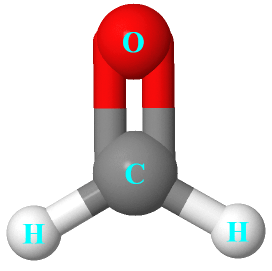Formaldehyde is the simplest of a class of functional groups called Aldehydes. It is an organic compound with the chemical formula H2CO. It is the simplest form of aldehyde compromised of one oxygen, one carbon, and two hydrogen atom.

In this tutorial, we will discuss H2CO lewis structure, molecular geometry, polar or nonpolar, bond angle, hybridization, etc.

Formaldehyde is stored in aqueous solutions known as formalin and it is highly reactive, therefore, it is used in synthetic chemistry.

Properties of Formaldehyde

• It has a molar mass of 30.02 g·mol−1.
• It is a toxic gas with an irritating and pungent smell.
• It has a boiling point of -19 °C and a melting point of -92 °C.
• It is soluble in acetone as well as in aqueous solution
 Name of Molecule Formaldehyde Chemical formula H2CO Molecular geometry of H2CO Trigonal planar Electron geometry of H2CO Trigonal planar Hybridization Sp2 Nature Polar molecule Total Valence electron for H2CO 12
Page Contents

## How to draw lewis structure for H2CO

H2CO lewis’s structure is made up of one oxygen, one carbon, and two hydrogens. These atoms are structured in a way that, the carbon atom is kept in a central position and it is connected with two hydrogens with a single bond and double-bonded with an oxygen atom.

There are a total of 2 lone pairs and 4 bonded pairs(two single bonds + one double bond) present in the lewis structure of H2CO.

Let’s take a look at how to draw the lewis dot structure of H2CO with some simple steps.

## Follow some steps for drawing the lewis dot structure of H2CO

1. Count total valence electron in H2CO

As we know, the lewis diagram is all about representing the valence electron of atoms within the molecule. Valence electrons are the outermost electron of an atom that can participate in the bond formation either by donating or accepting.

So, in the first step, we will find the total valence electron of H2CO. To calculate this, look at the periodic group of carbon, hydrogen, and oxygen.

The carbon atom belongs to Group 4A or 14th in the periodic table, hence, it has 4 valence electrons. The oxygen atom belongs to Group 6A or 16, hence, it has a 6 valence electron whereas the hydrogen atom is situated at Group 1A, hence the number of the valence electrons in hydrogen is 1.

⇒ Total number of the valence electrons in carbon = 4

⇒ Total number of the valence electrons in oxygen = 6

⇒ Total number of the valence electrons in hydrogen = 1

∴ Total number of valence electrons available for drawing the Lewis structure of H2CO = 4 + 1(2) + 6 = 12 valence electrons         [∴H2CO molecule has one carbon, one oxygen, and two hydrogens]2. Find the least electronegative atom and placed it at center

This step can be tricky sometimes but in the case of the H2CO molecule, it is very easy to determine the least electronegative atoms for placing at the central position in the lewis diagram.

One should remember that the hydrogen atom can never take the position of central in the lewis diagram because it can have only a maximum of two valence electrons in its outer shell, hence, it can never be able to share more than two electrons if required.

As central position demands the atom which is more prone to share the electrons with other atoms. The electronegativity of the oxygen atom is 3.44 and for the carbon atom, it is 2.55.

Hence, put the carbon at central position whereas spread the oxygen and hydrogen atoms evenly around it.3. Connect outer atoms to central atom with a single bond

In this step, join together each outer atom(oxygen and hydrogen) to the central atom(carbon) with the help of a single bond.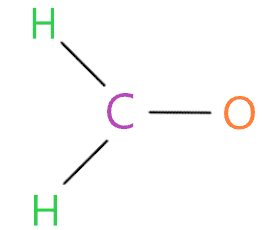Now we will count how many valence electrons we used in the above structure. In the above structure, we have used three single bonds for connecting two hydrogen atoms and one oxygen atom to the central atom(carbon).

A single bond means two electrons, hence, (3 single bonds × 2) = 6 valence electrons are used from a total of 12 valence electrons that are available for drawing the Lewis structure of H2CO.

∴ (12 – 6) = 6 valence electrons

So, we are left with 6 valence electrons more.

4. Place remaining valence electrons starting from outer atom first

Now we will start putting the remaining valence electrons to the outer atoms for completing their octet. In an H2CO molecule, the oxygen and hydrogen atoms are the outer atoms.

The hydrogen atom only needs two valence electrons to complete its octet and attains stability, moreover, the oxygen atom needs 8 electrons for completing the octet and coming in a stable form.

If you look at the 3rd step structure, both hydrogen atoms already getting the two electrons in their outer shell in form of a single bond, hence, both these already completed their octet.

So, just put the remaining valence electron over the oxygen atom till it’s complete the octet shell.So, by looking at the above structure, we see the oxygen atom has 8 electrons(6 electrons represented as dots + 2 electrons in a single bond connected to it) in its octet shell. Hence, it also completed its octet.

In the above structure, we have used a total of 12 valence electrons(three single bonds that contain 6 electrons and 6 electrons as dots).

Hence, now we have no valence electrons left.

5. Complete central atom octet and make covalent bond if necessary

The central atom in the H2CO molecule is carbon which also needs 8 electrons in its outer shell for completing the octet. In the 4th step structure, we see the carbon atom has 6 electrons in its outer shell in form of three single bonds but it needs 2 more electrons for completing the octet.

But the problem is we don’t have any remaining valence electrons, therefore, in this situation, we will convert the one lone pair of oxygen atoms into a single covalent bond.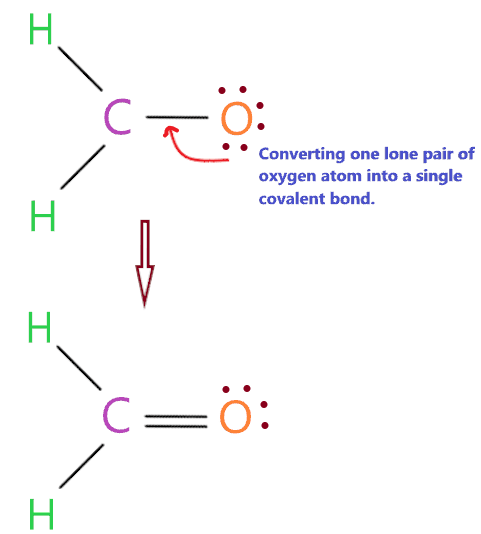As you see in the above structure, we have successfully converted the one lone pair of oxygen atoms to a covalent bond, hence, the carbon central also completed its octet as it has 8 electrons(two single bonds + one double bond) in its valence or outer shell.### H2CO lewis dot structure

That’s it, as we see in the above structure, all atoms(hydrogen, oxygen, and carbon) completed their octet.

Before, heading to the next part, we should check the stability of the above lewis diagram with the help of the formal charge concept.

6. Check the stability with the help of a formal charge concept

“The lesser the formal charge on atoms, the better is the stability of the lewis diagram.”

To calculate the formal charge on an atom. Use the formula given below-Let’s start with the central atom which is carbon in the H2CO molecule.

For carbon atom:

⇒ Valence electrons of carbon = 4

⇒ Lone pair electrons on carbon = 0

⇒ Shared pair electrons around carbon(two single bond and one double bond) = 8

∴ (4 – 0 – 8/2) = 0 formal charge on the carbon central atom.

For oxygen atom

⇒ Valence electrons of oxygen = 6

⇒ Lone pair electrons on oxygen = 4

⇒ Shared pair electrons around oxygen (1 double bond) = 4

∴ (6 – 4 – 4/2) = o formal charge on the oxygen atom.

For hydrogen atom

⇒ Valence electrons of hydrogen = 1

⇒ Lone pair electrons on hydrogen = 0

⇒ Shared pair electrons around hydrogen (1 single bond) = 2

∴ (1 – 0 – 2/2) = o formal charge on both hydrogen atoms.

So, each atom(oxygen, hydrogen, and carbon) in the H2CO lewis structure gets a formal charge equal to zero.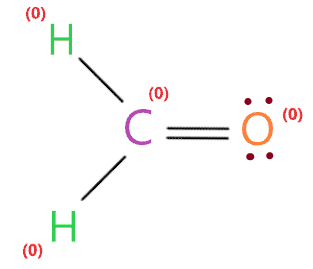Therefore, the above lewis structure of H2CO is most appropriate, reliable, and stable in nature because the overall formal charge is zero.

## What are the electron and molecular geometry of H2CO?

The molecular geometry of H2CO is trigonal planar as the carbon central atom has no lone pair and is attached to the two hydrogens atoms and one oxygen atom with the help of two single bonds and one double bond. So, there are three regions of electron density around the carbon central atom.

“A region of electron density means the group of bonding or nonbonding electrons that present around the atom. The single bond, double bond, or even triple bond around the atom will be counted as one region.”The electron pair around the carbon central atom will repel each other and tried to go far from each other, they will take the position where repulsion becomes minimum between them.

According to the VSEPR theory, the central atom with three regions of electron density adopts a trigonal planar geometry because repulsion is minimum in electron pairs at this position.

Hence, the final molecular geometry of H2CO appears as a trigonal planar.

The electron geometry of H2CO is also trigonal planar because there is no lone pair present on the central atom(carbon), hence, only bonded pair will be counted for determining the shape of H2CO.### H2CO molecular geometry

We can also find the electron and molecular geometry of H2CO using the AXN method and VSEPR chart.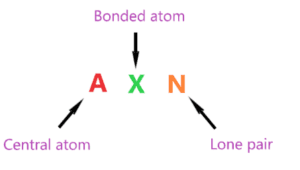AXN is a simple formula that represents the number of the bonded atom and lone pair on the central atom to predict the shape of the molecule using the VSEPR chart.

AXN notation for H2CO molecule:

• A denotes the central atom, so, carbon is the central atom in H2CO molecule A = Carbon
• X denotes the bonded atoms to the central atom, carbon is bonded with two hydrogen atoms and one oxygen atom. Therefore, X = 3
• N represents the lone pair on the central atom, as per H2CO lewis structure, the carbon central atom has zero lone pair. Hence, N = 0

So, the AXN generic formula for the H2CO molecule becomes AX3N0 or AX3.

As per the VSEPR chart, if a molecule gets AX3 generic formula then its molecular geometry will be trigonal planar and electron geometry will also be trigonal planar.

Look at the VSEPR chart below to clear your doubts.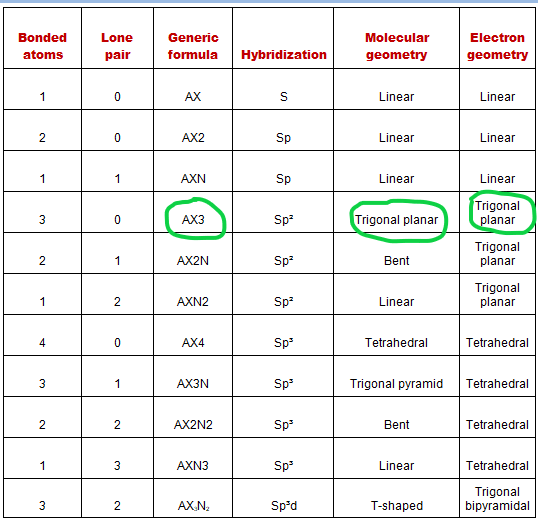## What is the Hybridization of H2CO?

“Hybridization is a theory that helps us understand the shape of molecular orbitals upon bonding to for compounds”

We will not go for the deep touch of the hybridization concept, we just want to know how to find the hybridization of the H2CO molecule.

Just catch the steric number of the H2CO molecule to gets it hybridized.

The steric number is simply an addition of bonded atom attached to the central atom and a lone pair present on the central atom.

∴ Steric number of H2CO = (Number of bonded atoms attached to carbon + Lone pair on carbon atom)

According to the lewis dot structure of H2CO, the carbon central atom is bonded with two hydrogen atoms and one oxygen atom and it contains no lone pairs.

∴ Steric number of H2CO = (3 + 0) = 3

 Steric number Hybridization 1 S 2 Sp 3 Sp² 4 Sp³ 5 Sp³d 6 Sp³d²

So, we get the Sp2 hybridization for the H2CO molecule because the steric number for the carbon central atom is 3.

“The process of intermixing of one S and two P orbitals to give three identical hybrid orbitals is known as Sp2 or trigonal hybridization. Sp2 has 33% s and 67% p character.”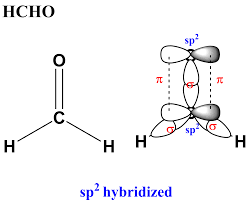## What is the bond angle of H2CO?

Every atom in the H2CO molecule has a bond angle of 120º with respect to the central atom because the central atom is surrounded by three regions of electron density and according to the VSEPR theory, these electron cloud needs to be far as possible to avoid the repulsive force.

The electron cloud formed the plane where the central atom(carbon) lies in the center of the triangle and other atoms are at the corner of the triangle. After, the bond will emerge from the central atom at angles of about 120° to each other.

The actual bond angles in the H2CO molecule are ∠HCH = 116 ° and ∠HCO = 122 °.## Formaldehyde polarity: is H2CO polar or nonpolar?

Well, we know the polar molecule has some dipole moment because of unequal distribution of charges whereas the non-polar molecule has an equal distribution of charges that cause zero dipole moment because they cancel out each other due to the symmetrical shape of the molecule.

Is H2CO polar or non-polar? H2CO is a polar molecule because of the difference in electronegativity value between carbon and oxygen that creates charge imbalance and generates some dipole moment in the molecule, makes it polar in nature.

Let’s understand why H2CO is polar in nature in detail.

## Two factors that indicate the polarity of H2CO

1. Electronegativity:

Electronegativity means the tendency of an atom to attracting electrons towards itself.

If the electronegativity difference between the atoms is high then the polarity will also be higher. Now, look at the electronegativity of carbon and oxygen.

As carbon electronegativity is around 2.6 and for oxygen, it is around 3.45. Therefore, oxygen has a higher tendency to attract an electron to itself than carbon.

Also, the electronegativity difference between carbon and oxygen is more than 0.5, and according to the Pauling scale if the electronegativity difference between atoms is higher than 0.5 then the bond formed between atoms behaves as polar.

2. Dipole moment:

Dipole moment ensures the strength of polarity between carbon and oxygen atom. As greater the dipole moment of the molecule, the more is the polar nature of that molecule. The electronegativity difference between the atoms(Carbon and oxygen) induced positive and negative charges.

As oxygen is more electronegative than carbon, hence, some negative charge is induced on the oxygen atom and a partial positive charge is induced on the carbon atom.

The inducing of these positive and negative charges generates the dipole moment directed from carbon to oxygen atom because oxygen atom is more electronegative than a carbon atom, so, it attracts more electrons towards itself.The net dipole moment of formaldehyde is 2.330 D.

In mathematical terms, we can express dipole moment as D = Q×R

Dipole moment formula = charge on the atoms * the distance between them.

## FAQ

### Why the double bond is formed between carbon and oxygen atom in the H2CO lewis structure?

This is because the carbon needs 8 electrons in its outer shell to complete the octet and attains stability, hence, for completing its octet, we converted one lone pair of oxygen atoms to a single covalent bond.

Therefore, the bond formed between carbon and oxygen in the H2CO molecule is a double covalent bond in nature.

Also, the formal charge formed on double-bonded carbon and oxygen atom is completely neutralized, hence, it ensures the stability of the H2CO lewis dot structure.

### How many shared pair or lone pair electrons are present in the H2CO lewis structure?

Shared pairs electrons are the electrons that take part in the formation of the chemical bond between two atoms and lone pair electrons are the electrons that do not take part in any form of bond between two atoms.

As per H2CO lewis structure, the carbon central atom is attached with two single bonds and one double bond, hence, the number of shared pair electrons are (2 single bond×2 + 1 double bond ×4) = 8 shared pair of electrons.

Also, there are only four lone pairs of electrons are present on oxygen atoms and zero on the carbon and hydrogen atoms in the H2CO lewis dot structure.

### Why are the electron and molecular geometry of H2CO is same?

Electron geometry predicts the geometry of a molecule with the help of lone pair as well bond pair but molecular geometry has only taken bond pair to predict the shape of the molecule.

As we know, there is no lone pair present on the central atom(carbon) in the H2CO molecule, hence, the prediction of the geometry is only done on the basis of bonded pair around the central atom.

Therefore, the both electron and molecular geometry of H2CO is the same which is trigonal planar in nature.

## Summary

• The total valence electron is available for drawing the H2CO lewis structure is 12.
• The actual bond angles in the H2CO molecule are ∠HCH = 116 ° and ∠HCO = 122 °.
• H2CO is a polar molecule because of the difference in electronegativity value between carbon and oxygen that creates charge imbalance and generates some dipole moment in the molecule, makes it polar in nature.
• There are a total of 4 lone pairs of electrons and 8 shared pairs of electrons present in the lewis dot structure of H2CO.
• The electron geometry, as well as molecular geometry of H2CO, is trigonal planar as per VSEPR theory.
• The net dipole moment of formaldehyde is 2.330 D.
• The steric number for the carbon central atom is 3, hence, the Sp2 hybridization types formed in the H2CO molecule.
##### Subscribe to Blog via Email

Join 2 other subscribers

Share it...